## General Question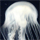# What is the name of the theorem that proves this equation?

Asked by abh94 (62) November 8th, 2009

The equation is as follows:

a^3—b^3 = 3(b^2) + 3b + 1
ex) 10^3—9^3 = 3(9^2) + 3(9) + 1 = 271

I saw it used on a math video. I am interested in how it is proved.

Observing members: 0Composing members: 0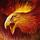More information is needed. As it stands, I can think of several examples where it isn’t true.

a = 0, b = 0: 0 – 0 ≠ 0 + 0 + 1
a = 0, b = 1: 0 – 1 ≠ 3 + 3 + 1

phoenyx (7388)“Great Answer” (0) Flag as…the only restriction is a>b and that a and b are positive integers

abh94 (62)“Great Answer” (0) Flag as…a = 1, b = -1: 1 + 1 ≠ 3 – 3 + 1
a = 1.5, b = 1: 3.375 – 1 ≠ 3 + 3 + 1

Perhaps a and b both have to be positive integers?

phoenyx (7388)“Great Answer” (0) Flag as…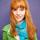I’ve never seen this but it’s a little bit similar to this
(a+b)^2=a^2+2ab+b^2
As you probaly know this can be used when is (a-b)^2 or when there are more than 2 terms

So you should check this maybe your equations is a derivate of this one

Christian95 (3260)“Great Answer” (0) Flag as…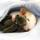The Binomial Theorem, according to which: (b + 1)^3 = b^3 + 3b^2 +3b +1.

Therefore a^3—b^3 = 3b^2 + 3b + 1 if and only if a = b + 1.

ratboy (15167)“Great Answer” (3) Flag as…The equation as you have written it is incorrect. Notice that there is no a term on the right side. It works for a=10 and b=9, but will not be true in general. Try using a=2 and b=1.

For any value of b, there are three values of a (two of which may be complex) that will be solutions. There is a formula for this, similar to to the quadratic equation, but I am too lazy to look it up just now.

(a^3 – b^3) can be factored as
(a^3 – b^3) = (a – b)(a^2 + ab – b^2)

LostInParadise (31284)“Great Answer” (1) Flag as…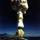@ratboy You nailed it! But what use is the relationship, practically. Maybe used in programming algorithms for incrementation functionality ? It certainly does not have to have ANY use. <g>

virtualist (2442)“Great Answer” (0) Flag as…or